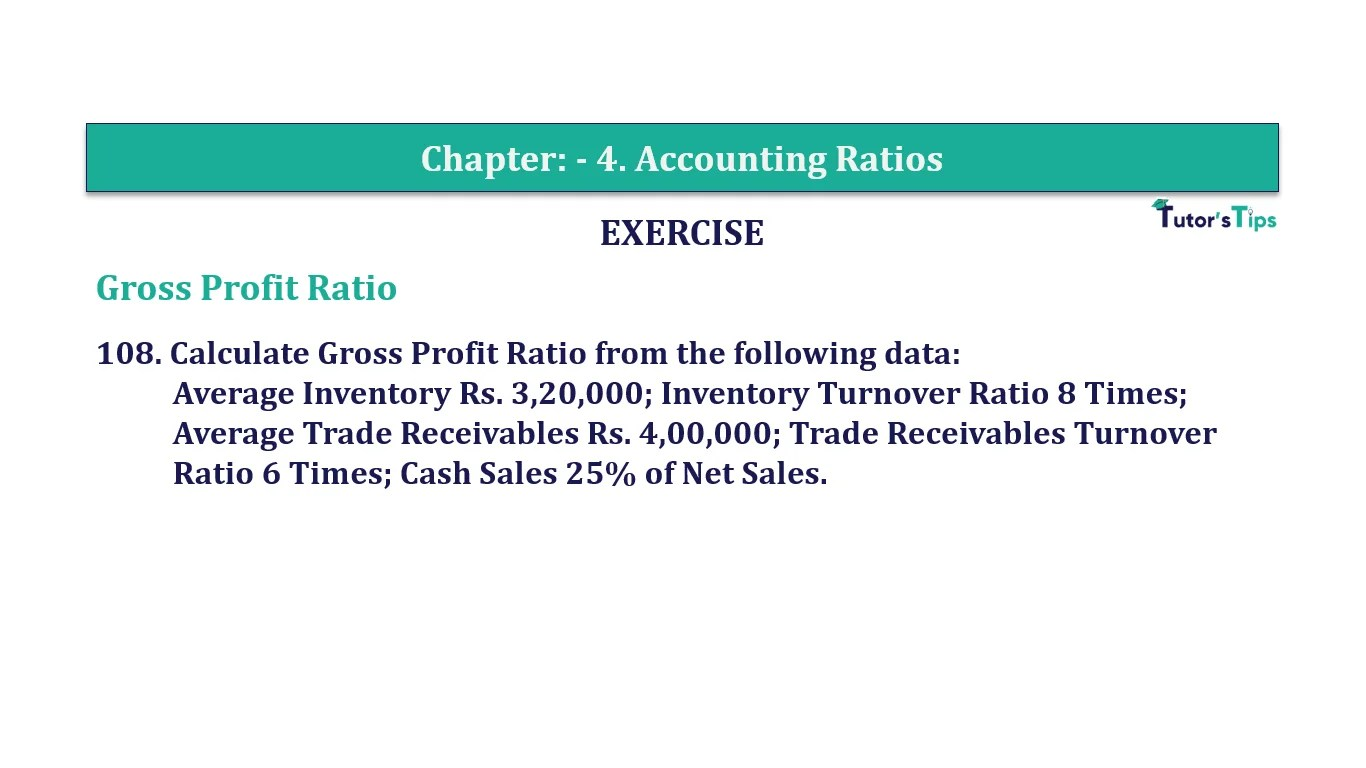# Question 108 Chapter 4 of +2-B – T.S. Grewal 12 ClassQuestion No. 108- Chapter No.4 - T.S. Grewal +2 Book Part B

Question 108 Chapter 4 of +2-B

Gross Profit Ratio

108. Calculate Gross Profit Ratio from the following data:
Average Inventory Rs. 3,20,000; Inventory Turnover Ratio 8 Times;
Ratio 6 Times; Cash Sales 25% of Net Sales.

### The solution of Question 108 Chapter 4 of +2-B: –

 Inventory Turnover Ratio = 8 Times Average Inventory = Rs 3,20,000

 Inventory Turnover Ratio = Cost of Goods Sold Average Inventory
 8 = Cost of Goods Sold Rs. 3,20,000 = 3,20,000 x 8 Cost of Goods Sold = Rs. 25,60,000

 Trade Receivables Turnover Ratio = 6 Times Average Trade Receivables = Rs. 4,00,000
 6 = Net Credit Sales Rs. 4,00,000 Net Credit Sales = Rs. 4,00,000 x 6 = Rs. 24,00,000 Total Sales = Cash Sales + Credit Sales Total Sales = 25% of Total Sales + Credit Sales 75% of Total Sales = Rs 24,00,000
 Total Sales = Rs. 24,00,000 75% = Rs 32,00,000 Gross Profit = Total Sales – Cost of Goods Sold = Rs. 32,00,000 – Rs. 25,60,000 = Rs. 6,40,000

 Revenue from Operations = Gross Profit X 100 Net Sales
 Revenue from Operations = Rs. 6,40,000 X 100 Rs.32,00,000 = 20%

Balance Sheet: Meaning, Format & Examples

Comment if you have any question.

Also, Check out the solved question of previous Chapters: –